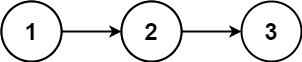# GeetCode Hub

Given a singly linked list, return a random node's value from the linked list. Each node must have the same probability of being chosen.

Example 1:```Input
["Solution", "getRandom", "getRandom", "getRandom", "getRandom", "getRandom"]
[[[1, 2, 3]], [], [], [], [], []]
Output
[null, 1, 3, 2, 2, 3]

Explanation
Solution solution = new Solution([1, 2, 3]);
solution.getRandom(); // return 1
solution.getRandom(); // return 3
solution.getRandom(); // return 2
solution.getRandom(); // return 2
solution.getRandom(); // return 3
// getRandom() should return either 1, 2, or 3 randomly. Each element should have equal probability of returning.
```

Constraints:

• The number of nodes in the linked list will be in the range `[1, 104]`.
• `-104 <= Node.val <= 104`
• At most `104` calls will be made to `getRandom`.